CONIC SECTIONS: THE HYPERBOLA

Example 1:

Find the coordinates of the center, vertices, and foci of the hyperbola given by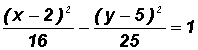. The equation is already in standard form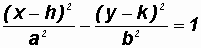where h = 2, k = 5, a = 4 (from denominator of positive term!), and b = 5

Now, we can find the requested information as follows:

Coordinates of the Center (h, k)

(2, 5)

Coordinates of the Vertices (h + a, k) and (h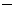a, k)

(2 + 4, 5) and (24, 5)

then (6, 5) and (2, 5)

Coordinates of the Foci (h + c, k) and (hc, k), whereSince a = 4 and b = 5, then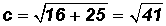.and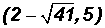Example 2:

Find the coordinates of the center, vertices, and foci of the hyperbola given by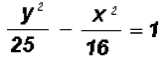.

First, we will convert the equation of the hyperbola to standard form. Since there is a positive y2-term raised to the second power we know that this hyperbola is of the form.

NOTE: The center (h, k) of this hyperbola is at (0, 0) because there is only a y2-term and an x2-term. Therefore, we can writewhere h = 0, k = 0, a = 5 (from denominator of positive term!), and b = 4

Now, we can find the requested information as follows:

Coordinates of the Center (h, k)

(0, 0)

Coordinates of the Vertices (h, k + a) and (h, ka)

(0, 0 + 5) and (0, 05)

then (0, 5) and (0,5)

Coordinates of the Foci (h, k + c) and (h, kc), whereSince a = 5 and b = 4, then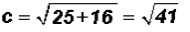.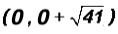and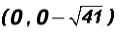thenand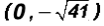Example 3:

Find the coordinates of the center, vertices, foci, and the equations of the asymptotes of the hyperbola given by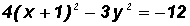.

First, we will convert the equation of the hyperbola to standard form.

It is eitheror, where a = b or a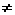b

Let's divide both sides of the equation by12 to get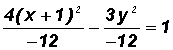and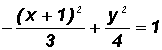or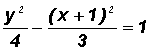We can now write the standard form of the given hyperbolic equation as follows:where h =1, k = 0, a = 2 (from denominator of positive term!), and b =Now, we can find the requested information as follows:

Coordinates of the Center (h, k)

(1, 0)

Coordinates of the Vertices (h, k + a) and (h, ka)

(1, 0 + 2) and (1, 02)

(1, 2) and (1,2)

Coordinates of the Foci (h, k + c) and (h, kc), whereSince a = 2 and b =, then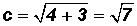.and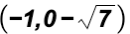and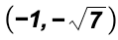Example 4:

Find the coordinates of the center, vertices, and foci of the hyperbola given by.

First, we will convert the equation of the hyperbola to standard form. Since there is a positive x2-term raised to the second power we know that this hyperbola is of the form.

The given equation is almost in standard form. All we have to do is give the x2-term a denominator of 1 as follows: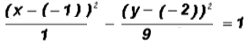where h = 1, k = 2, a = 1 (from denominator of positive term!), and b = 3

Now, we can find the requested information as follows:

Coordinates of the Center (h, k)

(1, 2)

Coordinates of the Vertices (h + a, k) and (ha, k)

(1 + 1, 2) and (11, 2)

then (2, 2) and (0, 2)

Coordinates of the Foci (h + c, k) and (hc, k), whereSince a = 1 and b = 3, then.

(1 + 2, 2) and (12, 2)

then (3, 2) and (1, 2)